# 对象“变形记”——初识引用与GC | 带你学《Java面向对象编程》之三

【本节目标】

## 3.1　引用传递分析

public class JavaDemo {
public static void main(String args[]) {
Person per1 = new Person() ;     //声明并实例化对象
per1.name = “张三” ;
per1.age = 18 ;
Person per2 = per1 ; //引用传递
per2.age =80 ;
per1.tell() ;            //进行方法的调用
}
}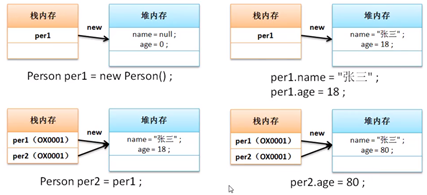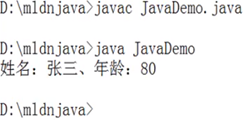public class JavaDemo {
public static void main(String args[]) {
Person per = new Person() ;     //声明并实例化对象
per.name = “张三” ;
per.age = 18 ;
change(per) ;     //等价于：Person temp = per ;
per.tell() ;            //进行方法的调用
}
public static void change(Person  temp){
temp.age = 80 ;
}
}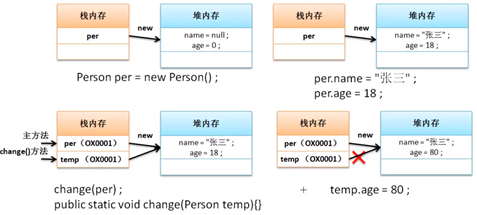## 3.2　引用与垃圾产生分析

public class JavaDemo {
public static void main(String args[]) {
Person per1 = new Person() ;     //声明并实例化对象
Person per2 = new Person() ;
per1.name = “张三” ;
per1.age = 18 ;
per2.name = “李四” ;
per2.age =19 ;
per2 = per1 ;         //引用传递
per2.age =80 ;
per1.tell() ;            //进行方法的调用
}
}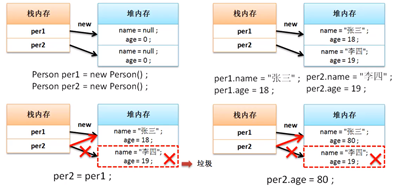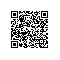Java开发者成长课堂